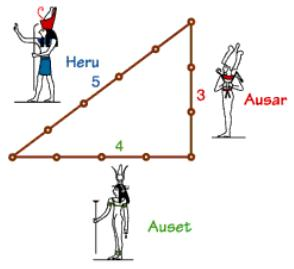Competitions

# EgyptLong time ago, the Egyptians figured out that a triangle with sides of length 3, 4 and 5 had a right angle as its largest angle. You must determine if other triangles have a similar property.

#### Input

Consists of several test cases, followed by a line containing 0 0 0. Each test case has three positive integers, less than 30000, denoting the lengths of the sides of a triangle.

#### Output

For each test case print a line containing "right" if the triangle is a right triangle, and a line containing "wrong" if the triangle is not a right triangle.

Time limit 1 second
Memory limit 128 MiB
Input example #1
6 8 10
25 52 60
5 12 13
0 0 0

Output example #1
right
wrong
right